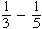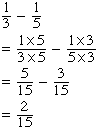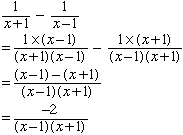Adding and subtracting Rational expressions. I am in grade 10 and I am a student here is an example of the questions: 1/(x+1) - 1/(x-1) = ? thanks for you help Hi Donna, Adding and subtracting rational exprressions is very similar to adding and subtracting fractions. Suppose you haveYou first need to find a common denominator. For denominators of 3 and 5 a common denominator is 3 x 5 = 15. Thus for one-third multiply the numerator and denominator by 5 and for one-fifth multiply the numerator and denominator by 3. That is:Likewise for denominators of x + 1 and x - 1 a common denominator is (x + 1) times (x - 1). Thus multiply the numerator and denominator of the first fraction by (x - 1) and multiply the numerator and denominator of the second fraction by (x + 1). You the getI hope this helps, Penny Go to Math Central